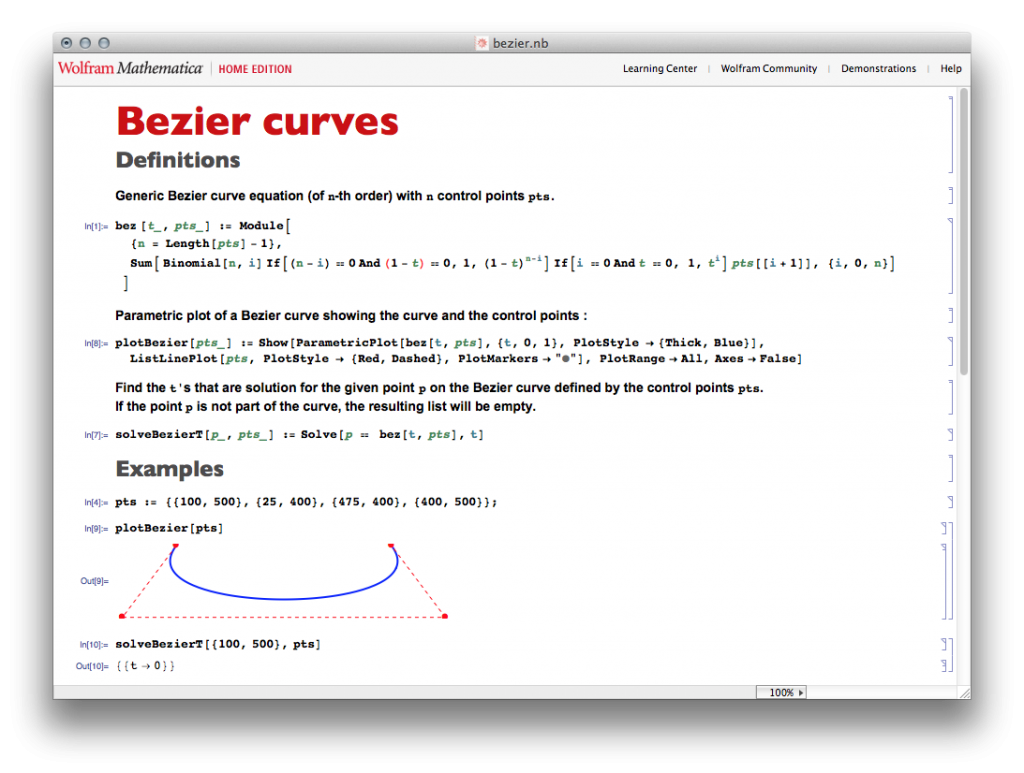# Explore Bezier Curves In Mathematica

As part of the next release of Inkscapemap I would like to introduce support for Bezier polylines, so I sketched up a Mathematica notebook to play around with a generic Bezier curve equation of n-th grade.

Mathematica already offers built-in Bezier curve plotting. However in order to understand the equation and code the solutions of the system `Px,y = beziern(t)` in Java, needed to calculate whether a point lies on a Bezier, I wanted to write the equation explicitly.

The Notebook allows for plotting and finding if a point lies on a curve. It is available on GitHub at marcoconti83/bezier-curves